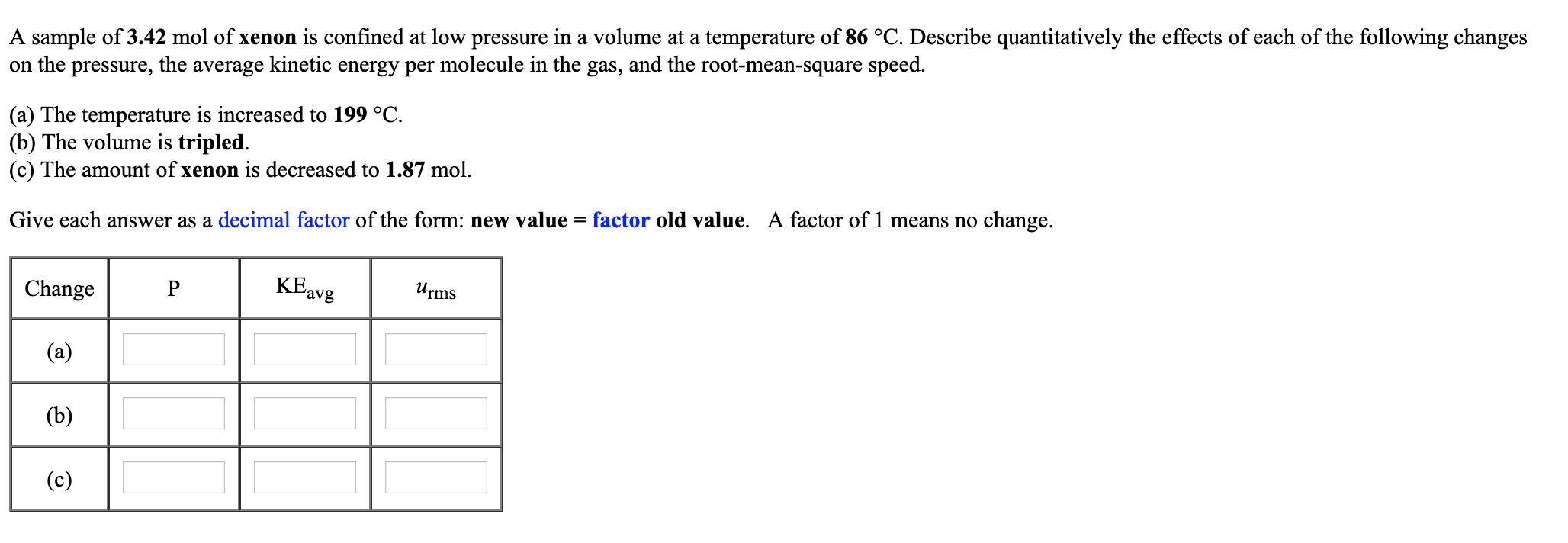# A sample of 3.42 mol of xenon is confined at low pressure in a volume at a temperature of 86 °C. Describe quantitatively the effects of each of the following changeson the pressure, the average kinetic energy per molecule in the gas, and the root-mean-square speed.(a) The temperature is increased to 199 °C(b) The volume is tripled.(c) The amount of xenon is decreased to 1.87 molGive each answer as a decimal factor of the form: new value factor old value. A factor of 1 means no change.ChangePKEavgms

Question
53 viewshelp_outlineImage TranscriptioncloseA sample of 3.42 mol of xenon is confined at low pressure in a volume at a temperature of 86 °C. Describe quantitatively the effects of each of the following changes on the pressure, the average kinetic energy per molecule in the gas, and the root-mean-square speed. (a) The temperature is increased to 199 °C (b) The volume is tripled. (c) The amount of xenon is decreased to 1.87 mol Give each answer as a decimal factor of the form: new value factor old value. A factor of 1 means no change. ChangeP KEavgms fullscreen
check_circle

Step 1

Given:

Step 2

Part a:

Step 3

Now,  KE avg is directly pr...

### Want to see the full answer?

See Solution

#### Want to see this answer and more?

Solutions are written by subject experts who are available 24/7. Questions are typically answered within 1 hour.*

See Solution
*Response times may vary by subject and question.
Tagged in

### Chemistry